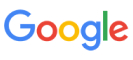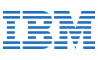New update is available. Click here to update.
Topics

# Optimal Strategy for a Game

Easy0/40
Average time to solve is 15m+6 more companies

## Problem statement

You and your friend Ninjax are playing a game of coins. Ninjax place the 'N' number of coins in a straight line.

The rule of the game is as follows:

``````1. Each coin has a value associated with it.

2. It’s a two-player game played against an opponent with alternating turns.

3. At each turn, the player picks either the first or the last coin from the line and permanently removes it.

4. The value associated with the coin picked by the player adds up to the total amount the player wins.
``````

Ninjax is a good friend of yours and asks you to start first.

Your task is to find the maximum amount you can definitely win at the end of this game.

Note:

``````'N' is always even number.

Ninjax is as smart as you, so he will play so as to maximize the amount he wins.
``````
Example 1:
``````Let the values associated with four coins be: [9, 5, 21, 7]

Let’s say that initially, you pick 9 and Ninjax picks 7.
Then, you pick 21 and Ninjax picks 5.
So, you win a total amount of (9+21), i.e. 30.

In case you would have picked up 7 initially and Ninjax would have picked 21 (as he plays optimally).
Then, you would pick 9 and Ninjax would choose 5. So, you win a total amount of (7+9), i.e. 16, which is not the maximum you can obtain.

Thus, the maximum amount you can win is 30.
``````
Example 2:
``````Let the values associated with four coins be: [20, 50, 5, 10]

Let’s say that initially, you pick 10 and Ninjax picks 20.
Then, you pick 50 and Ninjax picks 5.
So, you win a total amount of (10+50), i.e. 60.

In case you would have picked up 20 initially and Ninjax would have picked 50 (as he plays optimally).
Then, you would pick 10 and Ninjax would choose 5. So, you win a total amount of (20+10), i.e. 30, which is not the maximum you can obtain.

Thus, the maximum amount you can win is 60.
``````
Detailed explanation ( Input/output format, Notes, Images )
Constraints:
``````1 <= 'T' <= 10
2 <= 'N' <= 10 ^ 3
0 <= 'VALUE' <= 10 ^ 5

Where 'T' is the number of test cases, 'N' is the number of coins and 'VALUE' is the amount on each coin.

Time Limit: 1 sec
``````
Sample Input 1:
``````2
2
7 8
4
5 30 4 1
``````
Sample Output 1:
``````8
31
``````
Explanation For Sample Input 1:
``````For the first test case, you can pick the maximum value between 7 and 8, which is 8. Thus, Ninjax has to pick up 7.

So, you win a total amount of 8.
For the second test case, first, you pick 1, Ninjax picks 5. Then, you pick 30 and Ninjax picks 4, which is the only coin left. So, you win a total amount of (1 + 30) 31.
``````
Sample Input 2:
``````3
4
0 5 1 7
6
1 2 3 4 5 6
4
5 5 5 5
``````
Sample Output 2:
``````12
12
10
``````Console